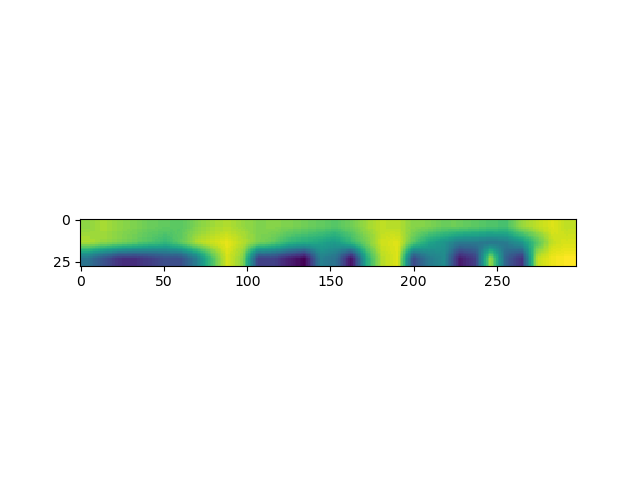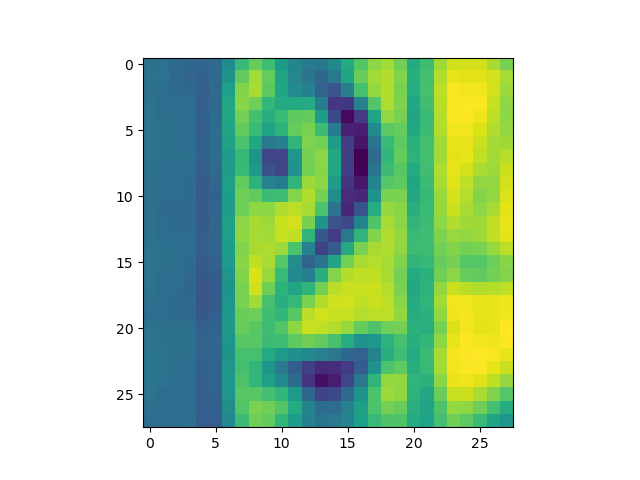# SVHN data grayscale and resize

Hi, sorry for yet another SVHN grayscale and resize problem permutation. After following lots of advice here on the forum I have a solution that renders some output. However when plotting a sample image it just shows a distorted color image, instead of the expected grayscale house number.

EDIT: I want a SVHN dataset with images in grayscale and size 28 x 28 in order to train the dataset on my MNIST CNN routine. Is this a correct method for fetching the dataset?

``````import torch
import torchvision
import torchvision.transforms as transforms
import torchvision.datasets as datasets
import matplotlib.pyplot as plt

class GetDataset(datasets.SVHN):
def __init__(self, root, split='train',
super(GetDataset, self).__init__(

def __getitem__(self, index):
"""
Args:
index (int): Index
Returns:
tuple: (image, target) where target is index of the target class.
"""
img, target = self.data[index], int(self.labels[index])

if self.transform is not None:
img = self.transform(img)

if self.target_transform is not None:
target = self.target_transform(target)

return img, target

svhn_transform = transforms.Compose([
transforms.ToPILImage(),
transforms.Resize((28, 28)),
transforms.Grayscale(num_output_channels=1),
transforms.ToTensor(),
# transforms.Normalize((0.5, 0.5), (0.5, 0.5))
transforms.Normalize([0.5], [0.5])
])

def main():
svhn_training_dataset = GetDataset(
root='./data',
transform=svhn_transform
)
batch_size=128,
shuffle=True,
num_workers=1)
plt.imshow(test_img[0, 0], cmap='gray')
plt.show()

if __name__ == '__main__':
main()
``````
Summary

This text will be hidden

After a bit of fiddling I find that setting `transforms.Resize(28)` returns an image of shape `(28, 298)`. Could my use of the `Grayscale()` function be wrong?You are missing the permutation inside your `__getitem__`:

``````img, target = self.data[index], int(self.labels[index])
img = np.transpose(img, (1, 2, 0))
if self.transform is not None:
...
``````

Since you are loading each sample as a numpy array, you would have to permute it manually.

1 Like

@ptrblck thanks so much for reaching out! Your answer certainly solved the dimensional weirdness.

Unfortunately there is still an issue with the grayscale. A sample image `test_img` has the shape `(1, 28, 28)`. If i try `plt.imshow(test_img.permute(1, 2, 0)` that is one dimension too many for `imshow()`. If I try `plt.imshow(test_img.squeeze()` I get the following picture. That sample image seem neither grayscaled nor appropriately resized. Any ideas?`matplotlib` uses the `viridis` colormap by default.
In your first code snippet you were setting the color map to `gray` via:

``````plt.imshow(test_img[0, 0], cmap='gray')
``````

@ptrblck sorry for that, of course you are right. I also realize that I was fooled by the high resolution of my screen. Thank you so much for helping, solution accepted.

1 Like

Haha, there is no reason to be sorry.